# User:IssaRice/Linear algebra/List of matrix products

Factorization theorems etc.

## Change of coordinate decompositions

The following decompositions can all be seen as a "simple" matrix sandwiched by two change of coordinate matrices, or equivalently as a "simple" matrix found by looking at it from the "right" bases.

Product name Matrix notation Change of coordinate matrix Linear transformation/basis notation Notes
A generic decomposition (as far as I know, this doesn't have a name)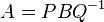$A = PBQ^{-1}$, where$P$ and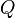$Q$ are invertible matrices$[T]_\sigma^\sigma = [I]_\beta^\sigma [T]_\alpha^\beta [I]_\sigma^\alpha = [I]_\beta^\sigma [T]_\alpha^\beta ([I]_\alpha^\sigma)^{-1}$ Many of the other decompositions can be seen as restrictions of this case: e.g. restricting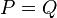$P = Q$, or restricting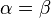$\alpha = \beta$, or restricting$\alpha,\beta$ to be orthonormal (in which case inverse and transpose (in the real case) and adjoint (in complex case) are the inverse).$A$ is diagonalizable; this is also called the eigendecomposition/spectral decomposition of$A$$A = QDQ^{-1}$ where$Q$ is invertible and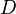$D$ is diagonal$[T]_\sigma^\sigma = [I]_\beta^\sigma[T]_\beta^\beta [I]_\sigma^\beta = [I]_\beta^\sigma[T]_\beta^\beta ([I]_\beta^\sigma)^{-1}$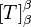$[T]_\beta^\beta$ is a diagonal matrix tao notes, p. 167, 168.$A$ is similar to$B$ / Relationship of the descriptions of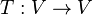$T : V \to V$ in one basis vs another$A = QBQ^{-1}$ where$Q$ is invertible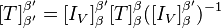$[T]_{\beta'}^{\beta'} = [I_V]_\beta^{\beta'} [T]_\beta^\beta ([I_V]_\beta^{\beta'})^{-1}$ see Tao notes p. 120
Singular value decomposition$A = U\Sigma V^*$, where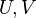$U,V$ are unitary,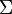$\Sigma$ is diagonal, and$V^*$ is the conjugate transpose of$V$$[T]_\sigma^\sigma = [I]_\beta^\sigma [T]_\alpha^\beta [I]_\sigma^\alpha$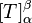$[T]_\alpha^\beta$ is a diagonal matrix, where$\alpha$ and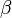$\beta$ are orthonormal bases
Schur decomposition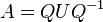$A = QUQ^{-1}$ where$Q$ is unitary and$U$ is upper triangular$[T]_\sigma^\sigma = [I]_\beta^\sigma [T]_\beta^\beta [I]_\sigma^\beta = [I]_\beta^\sigma [T]_\beta^\beta ([I]_\beta^\sigma)^{-1}$$[T]_\beta^\beta$ is upper triangular, where$\beta$ is an orthonormal basis

## Elementary operation decompositions

The following decompositions involve elementary operations. Since a matrix is invertible iff it is a product of elementary matrices iff it is a change of coordinate matrix, you might think elementary operation decompositions should somehow correspond to the change of coordinate decompositions from above. This sounds reasonable, but I haven't figured out the connection yet.

Product name Matrix notation Change of coordinate matrix Linear transformation/basis notation Notes
Inverting$A$ via elementary row operations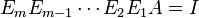$E_m E_{m-1} \cdots E_2 E_1 A = I$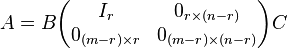$A = B\begin{pmatrix}I_r & 0_{r \times (n-r)} \\ 0_{(m-r)\times r} & 0_{(m-r)\times (n-r)}\end{pmatrix}C$ where$B$ and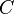$C$ are products of elementary matrices and$A$ has rank$r$ see tao notes p. 138